Function Repository Resource:

# IntegrateByParts

Get an inactive expression representing an integration by parts

Contributed by: Wolfram|Alpha Math Team
 ResourceFunction["IntegrateByParts"][f,x] returns the Inactive indefinite integration by parts of f with respect to x. ResourceFunction["IntegrateByParts"][f,{x,xmin,xmax}] returns the Inactive definite integration by parts of f with respect to x from xmin to xmax. ResourceFunction["IntegrateByParts"][f,x|{x,xmin,xmax},"Grid"] returns the Inactive integration by parts in a Grid highlighting the particular of u and dv that were used.

## Details and Options

Integration by parts is a technique for computing integrals, both definite and indefinite, that makes use of the chain rule for derivatives.
For an integral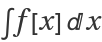, choose u and v such that f[x]x uv. Then, by computing u and integrating v to get v, we can write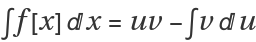.

## Examples

### Basic Examples (2)

Integrate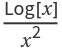by parts:

 In:=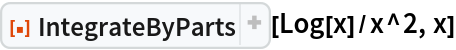Out=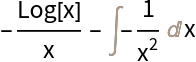Use Activate to evaluate the result:

 In:=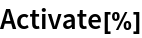Out=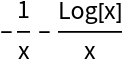Integrate xx by parts on the domain 0x1:

 In:=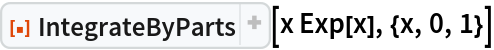Out=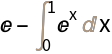Use Activate to fully evaluate the integral:

 In:=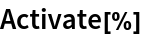Out=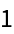### Scope (1)

To view the particular u and dv that were used to integrate by parts, use the optional third argument "Grid":

 In:=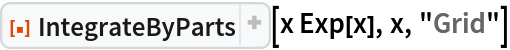Out=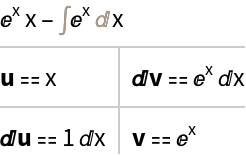### Options (2)

Use the option "ShowOtherDecompositions" to return a list of possible integrations by parts:

 In:=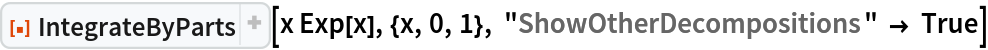Out=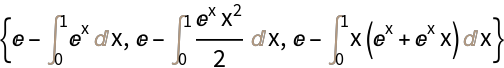The optional third argument "Grid" can be combined with the option "ShowOtherDecompositions":

 In:=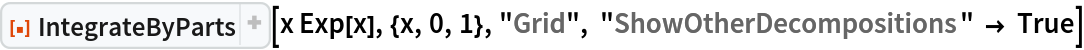Out=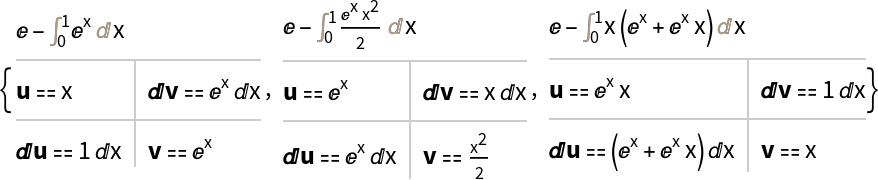### Applications (1)

Prove the reduction formula:

 In:=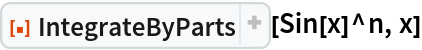Out=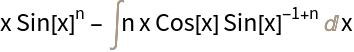### Possible Issues (2)

IntegrateByParts will return results, sometimes by the trivial decomposition uexpr and v⩵1ⅆx:

 In:=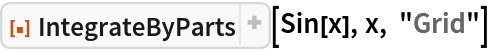Out=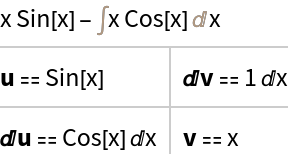If the given definite integral does not converge on the domain given, IntegrateByParts returns unevaluated with a message:

 In:=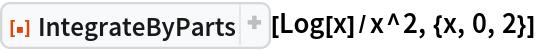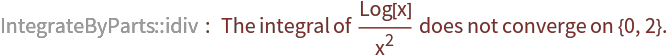Out=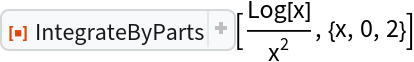### Neat Examples (6)

Compute an integral by integrating by parts twice:

 In:=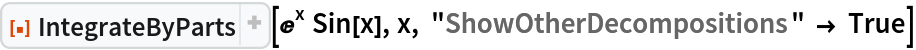Out=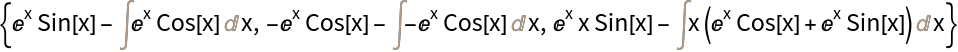Choose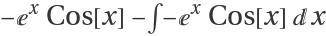and then integrate by parts again:

 In:=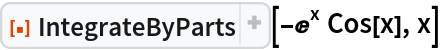Out=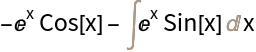Therefore we have:

==-x Cos[x]-==x Sin[x]-(-x Cos[x]-)

Which can be simplified to:

2* ==x (Sin[x]+Cos[x])

## Publisher

Wolfram|Alpha Math Team

## Version History

• 2.0.0 – 23 March 2023
• 1.0.0 – 01 April 2020